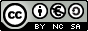First and Second Year of Secondary Education INDEX Introduction Objectives The square of a number and the square root. The mental calculation of the square root of a number The algorithm of the square root of a number The algorithm practice of the square root of a natural number The algorithm of the square root with decimal digits The algorithm practice of the square root with decimal digits Analysis of the algorithm of the square root
Square root
INTRODUCTION

After the four basic operations (addition, subtraction, multiplication and division) the square root is another mathematical operation which is traditionally studied at primary and secondary schools, because it is necessary to solve problems using
the Pythagoras theorem, proportions and second grade equations.

Nowadays, the calculation ease supplied by calculators and computers may lead us to think that learning mental calculation is no longer necessary. However, we also have technical means for writing at our disposal and, nonetheless, it is still necessary for us to learn how to write.

We consider a confident grasp of mental calculation to be a firm basis when solving any mathematical problem efficiently.

This unit revises the principles of square and square root, and shows the traditional algorithm to get the square root of a number.

OBJECTIVES
• To understand the principles of the square of a number and the square root of a number.
• To calculate mentally the square root of simple numbers.
• To learn and practise the algorithm to get the square root of a natural number.
• To learn and practise the algorithm to get the square root with decimal digits.
 Eduardo Barbero CorralSpanish Ministry of Education. Year 2007